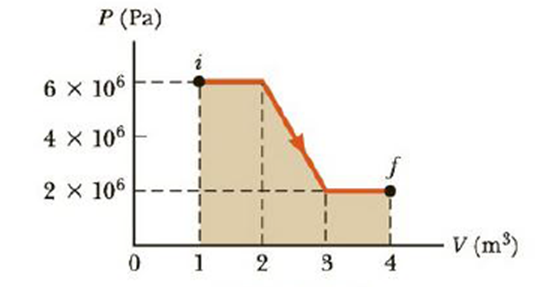Chapter 12, Problem 10P

Chapter
Section
Textbook Problem

(a) Determine the work done on a fluid that expands from i to f as indicated in Figure P12.10. (b) How much work is done on the fluid if it is compressed from f to i along the same path?Figure P12.10

(a)

To determine
the work done on the fluid that expands from i to f.

Explanation

Given Info:

The width of rectangle A is 3m3 .

The height of rectangle A is 2×106Pa .

The base of triangle C is 1m3 .

The height of triangle B is 4×106Pa .

The width of the rectangle B is 1m3 .

The height of the rectangle B is 6×106Pa .

The PV diagram of the fluid is,

The area under the graph in a PV diagram is equal in magnitude to the work done on the fluid. If the finial volume is greater than the initial volume, then the work done on the fluid is negative.

Formula to calculate the area is,

A=(W1×H1)+12(B2×H2)+(W3×H3)

• W1 is the width of rectangle A
• H1 is the height of rectangle A
• B2 is the base of triangle C
• H2 is the height of triangle C
• W3 is the width of rectangle B
• H3

(b)

To determine
The work done on the fluid that expands from f to i.

Still sussing out bartleby?

Check out a sample textbook solution.

See a sample solution

The Solution to Your Study Problems

Bartleby provides explanations to thousands of textbook problems written by our experts, many with advanced degrees!

Get Started

Find more solutions based on key concepts